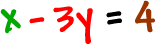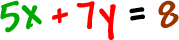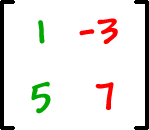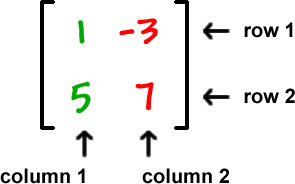You may or may not have seen this before -- it depends on where you took your last Algebra class.

First, I need to tell you about determinants.  We'll be using these to solve systems.

Say you've got a 2 x 2:We can make something called a "coefficient matrix":A matrix is just a grid of numbers with brackets around them.  (Remember that a coefficient is the number in front of the variable...  x = 1x, so the coefficient is 1.)  (We'll learn more about these matrix things in the next chapter.)

We've got rows and columns of the matrix: